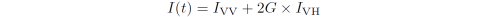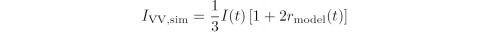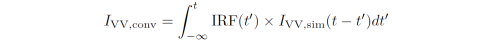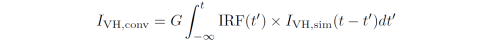### Fitting of time-resolved fluorescence anisotropy decays

AniFit implements a form of global direct vector reconvolution of the vertical and horizontal intensity decay components recorded in an anisotropy experiment in order to optimize the parameters of the anisotropy decay model. First the isotropic fluorophore intensity decayis optimized using iterative reconvolution with a multiexponential decay. The fitted sum, I(t), is then used as a constraint to simulate each of the VV and VH components:The simulated decays are convoluted with the instrument response function (IRF):and iteratively optimized to the measured decays by optimizing the parameter values in the theoretical expression for r(t) (the rotational model). This means that AniFit requires a single IRF only (usually VV and VH IRFs are identical).

### Analyse anisotropy decays in AniFit

AniFit can fit multiple data sets in one click.

1. Select the files you wish to analyse and their associated instrument response function in the data listboxes (note you can only specify one IRF at a time which will be used for all the selected decay data sets).

All of the selected decays and the IRF are plotted in the graph window.

2. Select the model to fit from the 'Fit model' listbox. In the MATLAB version of AniFit the fit model functions are located in the 'library/anisotropymodels' folder and can be modified at will. The mathematical expression of the model is displayed below the model listbox. In this example, the simplest anisotropy decay model is used, namely an exponential decay with a single rotational correlation time (one_rotation).

3. Specify the initial parameter value guesses and their lower and upper bounds in the parameter table. You can press the Plot button to plot a decay with the specified parameters.

Note that when multiple data sets are selected from the Decays listbox, it is the parameters of the first of the selected data sets that is being displayed in the table. To specify/see the parameters of the others data sets select these sets one by one. The parameter table is automatically updated according to the combination of selected decay, IRF and fit model.

4. Specify the number of exponentials used to fit the isotropic decay, i.e. I(t) = VV + 2G*VH (see first equation above). If you don't know this leave the value at 3 exponentials.

5. Specify whether to include the channel shift in between the IRF and the decays as a fitting parameter. The shift value will be optimized within the specified interval at a resolution of 1/Factor channels, where the Factor is specified in the Shift panel. A shift of 1 with a Factor of 10 thus corresponds to a shift of 1/10 channel.

6. Choose whether to include scatter in the fitting. In AniFit scatter is modelled by adding a fraction of the IRF to each of the convoluted decays (i.e. both I(t), VV(t) and VH(t)). The fraction of the scatter component is not the same for the different decays.

7. Choose whether to estimate the 95% confidence intervals (CI) of the fitted parameters using the Jacobian matrix of the fit. Estimating the CI from the Jacobian is not as accurate as calculating the full Chi-square surface. The confidence intervals will be displayed in the message board.

8. To run the fit press the Fit button or the green triangle in the Toolbar.

9. The fitted parameters are updated in the parameter table and the fits will be plotted in the graph window.

Again, if multiple data sets are selected from the Decays listbox only the optimized parameters of the first of the selected sets are being displayed in the table. In order to get all the information of the individual fits select the data sets one by one in the Decays listbox. The parameter table is updated according to the selection, the chi-square of the fit is shown in the top of the graph window, and the residuals are plotted above the graph.

If multiple data sets are selected the individual residuals can be plotted by pressing Ctrl+1.

10. A more informative evaluation of the fit is obtained by looking at the fitted VV and VH decays directly. Select the 'VV & VH' from the plot listbox. The plot now displays the individual VV and VH components and each of their residuals above the plot.

In this example above, the shift was not fitted resulting in a bad fit at early timescales. This is seen in the VV and VH plot but not in the r(t) plot. We fit the shift value by ticking the corresponding checkbox and run the fit again. The resulting fit with shift of 10 has a chi-square of 1.098 (compared to 2.998 without shift taken into account) and the individual VV and VH residuals are now highly improved: